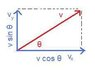# A few simple problems

highcontrast
I am taking an introductry physics course at the moment. I feel I have a grasp on the concepts. I was just wondering if anyone would care to check my work, and ensure I have the right answers.

Thanks

## Homework Statement

4. A diver in mexico drives off the top edge of a cliff 200m above, with a speed of 3m/s horiztonally. A) how long will it be before he hits the water below? b) where is the horiztonal distance from the foot of the cliff to the point where he enters the water? c) what are the horiztonal and vertical components of the divers velocity just as he enters the water?

All questions use 10m/s/s for gravitational accleration, except for #3, which uses 9.8m/s

## Homework Equations

As stated in attempts.

## The Attempt at a Solution

4 a) t^2 = d / 1/2A = 200 / 5 = 40 = root = 6.32S
b) 3m/s X 6.2s = 18.6M
c) I am not exactly sure what are the horizontal and vertical components, could anyone care to explain? i know triangles must be used. but I am having a difficult time understanding exactly what the question is asking for.. an angle? a speed? a distance?

Thank you again

Last edited:

godtripp
I didn't check everything, I'm on my way to bed. However on problem 2 part b they are asking for the distance at 3 seconds. In your equations you plugged in 4 seconds. An easy fix. Everything in part 1 is fine.

Haven't checked 3 or 4.

highcontrast
I have fixed the error, and confirmed that my answers are right for the other questions. However, I am still struggling with the horiztonal and vertical components question.

godtripp
on part b, you used 6.2 secs even though you calculated 6.32... why not use 6.32? or at least 6.3? Its erroneous to round down so far.

If I remember right all of your questions have had either horizontal or vertical initial velocities. Let's take for instance the case that we did not have such a convenient velocity. Instead the vector of velocity is "theta" degrees above the horizontal. Given this initial velocity and the angle, using trigonometry we can find the "x & y components" of that velocity. (See my sloppy picture I attached.)

These components of velocity is what we plug into our kinetic equations within projectile motion.

Now on to finding your components of velocity. The x component of velocity in this case is very easy to find. Since there is no acceleration in the x direction... the final velocity in the x component is equal to your initial (3 m/s).

Now plugging in your y components we can can find your y component of final velocity this is probably found most easily with the equation $$v^{2} = v_{0}^{2} + 2a(x-x_{0})$$

This gives us $$v=\sqrt{2*g*(200m)}$$
since your initial velocity in the y component is zero.

Hope I explained this enough, let me know if there's any more questions.

Also I just realized I used the terms "x & y" components when your question used horizontal and vertical. Hopefully this doesn't cause any confusion.

#### Attachments

•velocity.jpg
5.2 KB · Views: 334